### Weir slope

The weir slope is the downstream element of the boundary element pair defining a weir. The following constants have to be specified on the line beneath the *FLUID SECTION,TYPE=CHANNEL WEIR SLOPE card:

• the width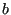• the slope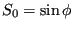(if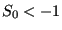the slope is calculated from the coordinates of the end nodes belonging to the element)
• the length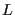(if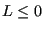the length is calculated from the coordinates of the end nodes belonging to the element)
• the trapezoid angle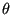• the grain diameter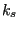for the White-Colebrook law or the Manning constant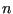for the Manning law (in the latter case the user has to specify the parameter MANNING on the *FLUID SECTION card)
• the number of the upstream weir crest element

The meaning ofandcan be derived from Figure 119. The value of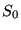is used to determine the critical depth on the crest. Moreover, it is used in the Bresse equation in case the flow is determined by the downstream conditions (inundated crest). It should simulate the mean slope in the absence of the weir. The lengthof the weir is shown in Figure 121.

Example files: channel7.